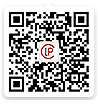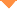首页 > 论文 > 中国激光 > 48卷 > 1期(pp：105002--1)

Periodic Evolution of Airy-Gaussian Beams Under Linear Potential

• 摘要• 论文信息• 参考文献• 被引情况• PDF全文Abstract

Objective Non-diffractive beam has been an important research topic in optics. In addition to Bessel beam which transmits along a straight line, Airy beam propagating along the quadratic parabola is also a special non-diffractive beam, and this is called self-acceleration property. And it also shows strong recovery force against disturbance during transmission, namely self-healing property. Airy-Gaussian beam, which can be adjusted between Airy beam and Gaussian beam via the distribution factor, is derived from Airy beam. Based on the nonlinear Schr?dinger equation, the transmission in different nonlinear media including the Kerr media, saturated media, and photorefractive media has been studied in recent years. Since Longhi introduced fractional Schr?dinger equation into the field of optics in 2015, it had aroused the interest of research groups at home and abroad. The study on Gaussian beam and Airy beam in the framework of fractional Schr?dinger equation has emerged in the endless stream, but there are few studies on Airy-Gaussian beam. In this paper, taking the fractional Schr?dinger equation as the theoretical model, the evolution process is simulated numerically by the split-step Fourier method to study the propagation and interaction of Airy-Gaussian beams with linear potential. The transmission of Airy-Gaussian beams under linear potential is explored to obtain some meaningful results about beam control. Our results provide theoretical reference for the applications in optical switching and optical logic devices as well as for the adjustment of beams in optical systems.

Methods The split-step Fourier method is a numerical method for solving nonlinear Schr?dinger equation, considering the diffraction effect and nonlinear effect separately over a small distance. In this paper, based on the fractional Schr?dinger equation, the propagation of single Airy-Gaussian beam and the interaction of dual Airy-Gaussian beams are simulated by the split-step Fourier method. When it comes to exploring the transmission of single Airy-Gaussian beam, the Lévy index, distribution factor, and linear coefficient are analyzed. As for the interaction of dual Airy-Gaussian beams, the effect of changing the Lévy index, beam interval as well as relative phase is observed.

Resultsand Discussion In the research process, it is found that Airy-Gaussian beam will split into two sub beams when there is no potential, the splitting phenomenon gradually disappears when there is a linear potential, and it evolves periodically, in which the energy of the main lobe hardly changes with the transmission distance and the same is to side lobes. The evolution performs differently when parameter values vary. The period is associated with linear coefficient β, performing that it becomes smaller when β is larger. Besides, the symbol of β can make a difference to the distribution space of the beam. That is when β>0, the beam is only distributed on the left side of the central axis, otherwise on the right side. It is worth mentioning that there is a transition process from splitting to non-splitting when β is closed to 0, in which one of the sub beams gradually approaches to the other, and they finally converge into a beam. When β>0, the incident beam deflects to the left, and vice versa to the right (Fig. 1). With the increase of Lévy index α from 1 to 2, the oscillation amplitude increases, and the evolution path changes from an approximate broken line to a curve. Furthermore, such oscillation is not symmetrical about the central axis, but extends in the half space along the central axis to the x axis (Fig. 2). The energy exchange occurs due to the competition when the energy difference is large, which happens when relative phase δ=±π/2. However, there is almost no competition in the case of in-phase or out-phase because the energy of two beams increases or decreases at the same time (Fig. 3). The intensity distribution changes from symmetry to asymmetry and then to symmetry with the increase of transmission distance in a period (Fig.4). When α exceeds a certain value, the energy exchange will be destroyed. In addition, when α becomes large, two beams do not propagate at equal intervals. At the beginning of any period, the interval between two beams is the largest and that at the half period is the smallest (Fig. 5).

Conclusion In this paper, a fractional Schr?dinger equation is used as the theoretical model to explore the propagation and interaction of Airy-Gaussian beams under linear potential by using the split-step Fourier method. Generally speaking, the linear potential will destroy the beam splitting, performing that when β increases from 0 to a very small range, and the beam will no longer split, but shows periodic evolution, which is not symmetrical about the central axis but distributed on one side of the central axis. And this evolution is affected by many parameters. Transverse oscillation amplitude and Lévy index α are positively correlated, performing that with the increase of α, the amplitude of transverse oscillation increases correspondingly due to the increment of diffraction effect, and the periodic evolution changes from an approximate broken line to a curve. Its period is affected by linear coefficient β, and it decreases with the increase of β. Moreover, the sign of β can change the deflection direction and distribution space of the beam. When β>0, the incident beam which only distributes on the left side of the central axis deflects to the left, and vice versa. In the process of the interaction, the energy of two beams will exchange periodically when δ=±π/2, but not in the in-phase and out-phase. In addition, this phenomenon will disappear when α approaches to 2. Furthermore, when α is large, two beams do not propagate at equal intervals, with the largest interval at the beginning of the period and the smallest at the half. These conclusions provide a theoretical reference for the application of beams in optical switching and optical logic devices as well as beam control.【1】Durnin J. Exact solutions for nondiffracting beams. I. The scalar theory [J]. Journal of the Optical Society of America A. 1987, 4(4): 651-654.Durnin J. Exact solutions for nondiffracting beams. I. The scalar theory [J]. Journal of the Optical Society of America A. 1987, 4(4): 651-654.

【2】Durnin J, Miceli J J, Eberly J H. Diffraction-free beams [J]. Physical Review Letters. 1987, 58(15): 1499-1501.

【3】Clerici M, Hu Y, Lassonde P, et al. Laser-assisted guiding of electric discharges around objects [J]. Science Advances. 2015, 1: e1400111.

【4】Liang Y, Hu Y, Song D, et al. Image signal transmission with Airy beams [J]. Optics Letters. 2015, 40(23): 5686-5689.

【5】Berry M V, Balazs N L. Nonspreading wave packets [J]. American Journal of Physics. 1979, 47(3): 264-267.

【6】Siviloglou G A, Broky J, Dogariu A, et al. Observation of accelerating Airy beams [J]. Physical Review Letters. 2007, 99(21): 213901.

【7】Siviloglou G A, Christodoulides D N. Accelerating finite energy Airy beams [J]. Optics Letters. 2007, 32(8): 979-981.

【8】Broky J, Siviloglou G A, Dogariu A, et al. Self-healing properties of optical Airy beams [J]. Optics Express. 2008, 16(17): 12880-12891.

【9】Siviloglou G A, Broky J, Dogariu A, et al. Ballistic dynamics of Airy beams [J]. Optics Letters. 2008, 33(3): 207-209.

【10】Bandres M A. Rodríguez-Lara B M. Nondiffracting accelerating waves: Weber waves and parabolic momentum [J]. New Journal of Physics. 2013, 15(1): 013054.

【11】Rose P, Diebel F, Boguslawski M, et al. Airy beam induced optical routing [J]. Applied Physics Letters. 2013, 102(10): 101101.

【12】Chen R P, Yin C F, Chu X X, et al. Effect of Kerr nonlinearity on an Airy beam [J]. Physical Review A. 2010, 82(4): 043832.

【13】Zhang Y Q, Beli? M R, Zhang H B, et al. Interactions of Airy beams, nonlinear accelerating beams, and induced solitons in Kerr and saturable nonlinear media [J]. Optics Express. 2014, 22(6): 7160-7171.

【16】Zhang L, Bai X Q, Wang Y H, et al. Interaction of Airy beams in a medium with parabolic potential [J]. Optik. 2018, 161: 106-110.

【18】Baumgartl J, Mazilu M, Dholakia K. Optically mediated particle clearing using Airy wavepackets [J]. Nature Photonics. 2008, 2(11): 675-678.

【19】Abdollahpour D, Suntsov S, Papazoglou D G, et al. Spatiotemporal Airy light bullets in the linear and nonlinear regimes [J]. Physical Review Letters. 2010, 105(25): 253901.

【20】Voloch-Bloch N, Lereah Y, Lilach Y, et al. Generation of electron Airy beams [J]. Nature. 2013, 494(7437): 331-335.

【21】Chen C D, Chen B, Peng X, et al. Propagation of Airy-Gaussian beam in Kerr medium [J]. Journal of Optics. 2015, 17(3): 035504.

【22】Zhou M L, Peng Y L, Chen C D, et al. Interaction of Airy-Gaussian beams in saturable media [J]. Chinese Physics B. 2016, 25(8): 084102.

【23】Chen W J, Song D, Li Y, et al. Control on interaction of Airy-Gaussian beams in competing nonlinear medium [J]. Acta Physica Sinica. 2019, 68(9): 094206.

【25】Bai X Q, Wang Y H, Zhang J, et al. Bound states of chirped Airy-Gaussian beams in a medium with a parabolic potential [J]. Applied Physics B. 2019, 125: 188.

【27】Laskin N. Fractional quantum mechanics [J]. Physical Review E. 2000, 62(3): 3135-3145.

【28】Laskin N. Fractional quantum mechanics and Lévy path integrals [J]. Physics Letters A. 2000, 268(4/5/6): 298-305.

【29】Laskin N. Fractional Schr?dinger equation [J]. Physical Review E. 2002, 66(5): 056108.

【30】Longhi S. Fractional Schr?dinger equation in optics [J]. Optics Letters. 2015, 40(6): 1117-1120.

【31】Zhang Y Q, Zhong H, Beli? M R, et al. Diffraction-free beams in fractional Schr?dinger equation [J]. Scientific Reports. 2016, 6: 23645.

【32】Zhang Y Q, Liu X, Beli? M R, et al. Propagation dynamics of a light beam in a fractional Schr?dinger equation [J]. Physical Review Letters. 2015, 115(18): 180403.

【33】Huang X W, Shi X H, Deng Z X, et al. Potential barrier-induced dynamics of finite energy Airy beams in fractional Schr?dinger equation [J]. Optics Express. 2017, 25(26): 32560-32569.

Xiao Yan,Zhang Jing,Wang Pengxiang. Periodic Evolution of Airy-Gaussian Beams Under Linear Potential[J]. Chinese Journal of Lasers, 2021, 48(1): 0105002